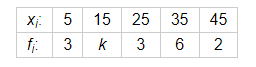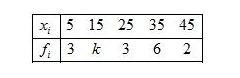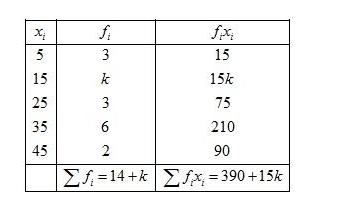# The arithmetic mean of the following data is 25,

Question:

The arithmetic mean of the following data is 25, find the value of k.Solution:

Given:Mean $=25$

First of all prepare the frequency table in such a way that its first column consist of the values of the variate $\left(x_{i}\right)$ and the second column the corresponding frequencies $\left(f_{i}\right)$.

Thereafter multiply the frequency of each row with corresponding values of variable to obtain third column containing $\left(f_{i} x_{i}\right)$.

Then, sum of all entries in the column second and denoted by $\sum f_{i}$ and in the third column to obtain $\sum f_{i} x_{i}$.We know that mean, $\bar{X}=\frac{\sum f_{i} x_{i}}{\sum f_{i}}$

$25=\frac{390+15 k}{14+k}$

By using cross multiplication method,

$350+25 k=390+15 k$

$25 k-15 k=390-350$

$10 k=40$

$k=4$

Hence, k = 4